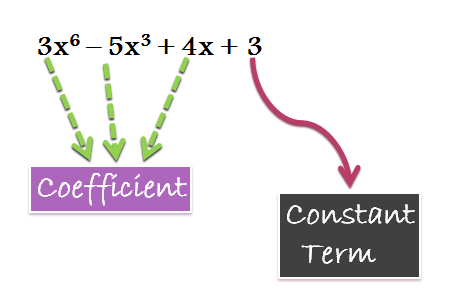## Algebra

#### FUNCTIONS-1

Theory of Equations: 1

Theory of Equations:

This lesson is the starting point of Algebra and deals with some very fundamental properties and theorems related to algebraic functions. So, we’ll study all such theorems and concepts in detail thereby to lay the foundation of algebra.

Polynomial:

Polynomial is derived from two words- poly meaning many and nomial means terms, so polynomial can be said to an algebraic expression involving many terms. Here one can witness the variables along with their exponents, their coefficients, mathematical operators like plus and minus and a constant term (not necessary). The polynomial function can be of a single variable or multiple variables.

e.g.Degree of a Polynomial:

The highest exponent of the variable in a polynomial is known as the degree of the polynomial. Eg. The degree of 4x5 -3x +2 is 5. Similarly the degree of  5x4 – 3x+x  is 4 .

Roots of a Polynomial Equation:

The roots of a polynomial, are the values of the variable that make it zero. In other words, the roots are those values of the variable at which the value of the polynomial becomes zero. It can also be said that roots are those value of x (if it is a single variable in x) at which the curve of the function cuts the X axis.

For example:  Consider a polynomial x2 – 11x + 24. This polynomial can be factorised as: x– 11x + 24 = (x-3)(x-8)

If we put x = 3 or x = 8, then the value of the polynomial becomes zero or we can say that x =3 and x=8 are the roots.